﻿ Some Integral Inequalities of Hermite-Hadamard Type for <i>ε</i>-convex FunctionsPublications are Open
Access in this journal
Article Versions
Export Article
• Normal Style
• MLA Style
• APA Style
• Chicago Style
Research Article
Open Access Peer-reviewed

### Some Integral Inequalities of Hermite-Hadamard Type for ε-convex Functions

Jun Zhang, Zhi-Li Pei, Feng Qi
Turkish Journal of Analysis and Number Theory. 2017, 5(3), 117-120. DOI: 10.12691/tjant-5-3-5
Received January 07, 2017; Revised May 04, 2017; Accepted May 19, 2017

### Abstract

In the paper, the authors establish a new integral identity. By this integral identity and Hölder’s inequality, the authors obtain some new inequalities of the Hermite-Hadamard type for ε-convex functions.

### 1. Introduction

We first list some definitions concerning various convex functions.

Definition 1.1. A function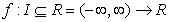is said to be convex if(1.1)

holds for all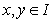and.

In 1 the concept of ε-convex functions was introduced as follows.

Definition 1.2. 1 A functionand, if(1.2)

is valid for alland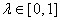, then we say thatis a ε-convex function on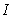.

The following inequalities of Hermite-Hadamard type were established for some of the above convex functions.

Theorem 1.1. 2 Let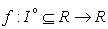be differentiable on,with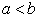.

(i) Ifis convex function on, then(1.3)

(ii) Ifis convex function on,, then(1.4)

Theorem 1.2. 3 Letbe differentiable on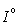,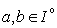withand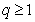.

(i) Ifis convex function on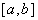, then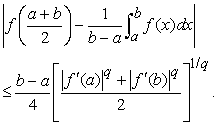(1.5)

(ii) Ifis convex function on,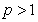, then(1.6)

and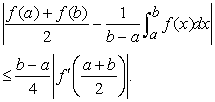(1.7)

In this paper, we establish a new integral identity. By this identity and Hölder’s inequality, some new Hermite-Hadamard type for the product of ε-convex function and discussed, some results are obtained.

### 2. Some Lemmas

Lemma 2.1 Letbe differentiable onand where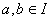with,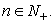If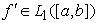, then the following identity holds:(2.1)

Proof.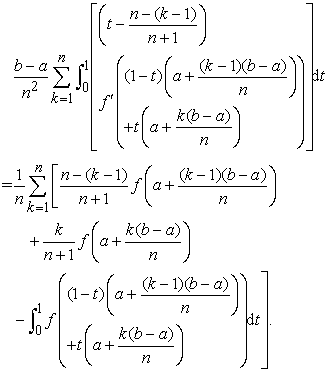(2.2)

Since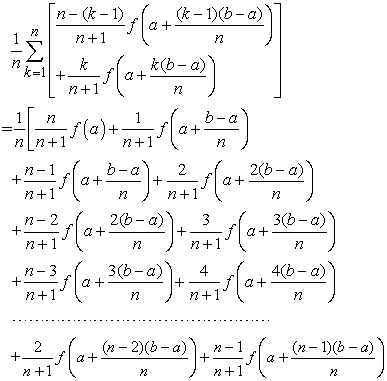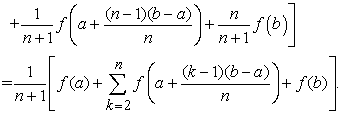(2.3)

Changing variables with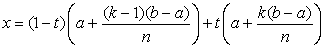for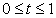, we get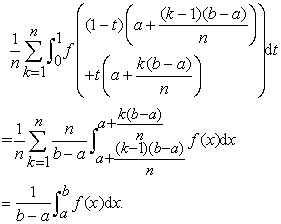(2.4)

Put the equalities (2.3) to (2.4) into the equality (2.2), the inequality (2.1) is thus proved. This completes of the proof.

By taking n= Lemma 2.1, we have the following identities.

Lemma 2.2 2 Letbe differentiable onand wherewith, if, then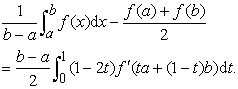### 3. Some Integral Inequalities of Hermite-Hadamard Type

Now we are in a position to establish some new integral inequalities of Hermite-Hadamard type involving the ε-convex functions.

Theorem 3.1 Let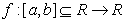be differentiable function onand,. If，then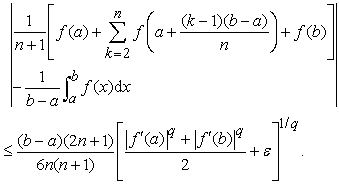(3.1)

Proof. Since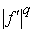is ε-convex function on, using the Lemma 2.1 and by the Hölder’s inequality, we have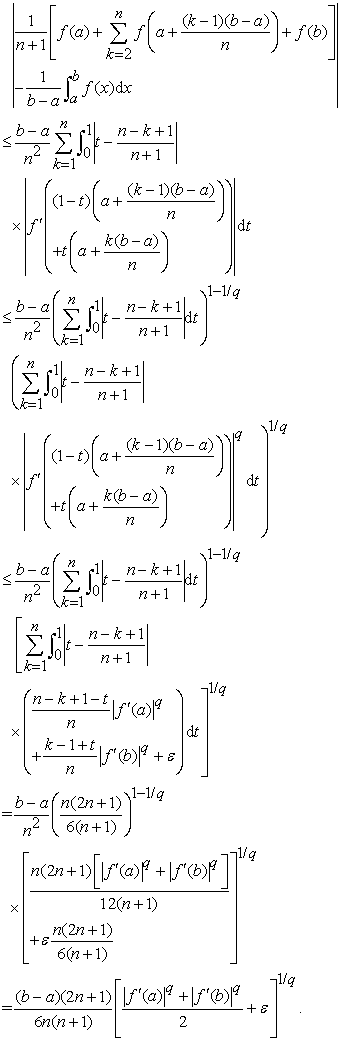Theorem 3.1 is proved.

Crollary3.1. Under the conditions of Theorem 3.1, then

(1) if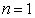, we have(2) if, we haveTheorem 3.2 Letbe differentiable function onand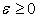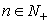,and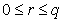. If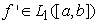，then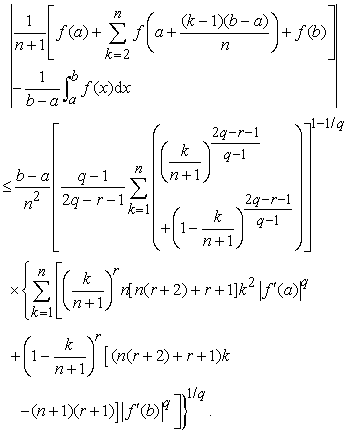(3.2)

Proof. Sinceis ε-convex function on, using the Lemma 2.1 and by the Hölder’s inequality, we have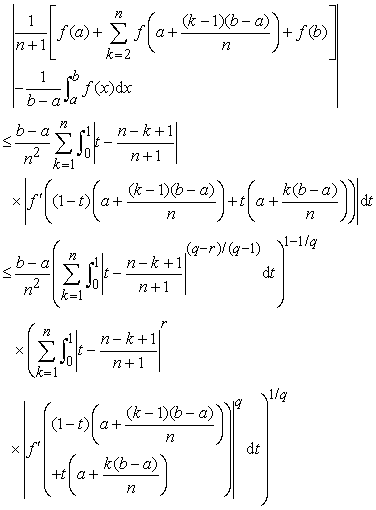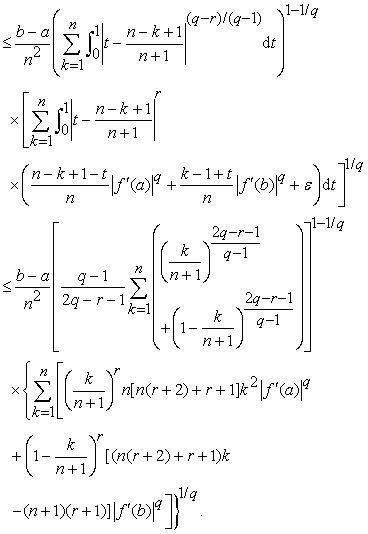(3.3)

Theorem 3.2 is proved.

### Acknowledgements

This work was supported by the National Natural Science Foundation under Grant No. 61373067 and No. 61672301 of China and by the Inner Mongolia Autonomous Region Natural Science Foundation Project under Grant No. 2015MS0123, China.

### References

  D. H. Hyers, S. M. Ulam, Approximately convex functions, Proc. Amer. Math. Soc., 3 (1952), 821-828. In article View Article  S. S. Dragomir, and R. P. Agarwal, Two inequalities for differentiable mappings and applications to special means of real numbers and to trapezoidal formula, Appl. Math. Lett., 11(1998), 91-95. In article View Article  C. E. M. Pearce and J. Pečarić, Inequalities for differentiable mappings with application to special means and quadrature formula. Appl. Math. Lett., 13(2000), 51–55. In article View Article  F. Qi and B.-Y. Xi, Some Hermite–Hadamard type inequalities for geometrically quasi-convex functions, Proceedings of the Indian Academy of Sciences (Mathematical Sciences), 124:3(2014), 333-342. In article View Article  F. Qi, T.-Y. Zhang, and B.-Y. Xi, Integral inequalities of Hermite-Hadamard type for functions whose first derivatives are of convexity, Ukrainian Mathematical Journal, 66:5(2015), 625-640. In article View Article  B.-Y. Xi, R.-F. Bai, and F. Qi, Hermite-Hadamard type inequalities for the m- and (α,m)-geometrically convex functions, Aequationes Math., 184:3(2012), 261-269. In article View Article  B.-Y. Xi and F. Qi, Hermite-Hadamard type inequalities for geometrically r-convex functions, Studia Scientiarum Mathematicarum Hungarica, 51:4(2014), 530-546;. In article View Article  B.-Y. Xi and F. Qi, Inequalities of Hermite-Hadamard type for extended s-convex functions and applications to means, J. Nonlinear Convex Anal,. 16(2015), 873-890. In article View Article  B.-Y. Xi, S.-H. Wang, and F. Qi, Some inequalities for (h,m)-convex functions, Journal of Inequalities and Applications, 2014, 100, 12~pages. In article View Article  B.-Y. Xi, T.-Y. Zhang, and F. Qi, Some inequalities of Hermite--Hadamard type for m-harmonic-arithmetically convex functions, ScienceAsia, 41: 5(2015), 357-361. In article View ArticleThis work is licensed under a Creative Commons Attribution 4.0 International License. To view a copy of this license, visit http://creativecommons.org/licenses/by/4.0/